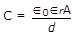# Electronics and Communication Engineering - Materials and Components

### Exercise :: Materials and Components - Section 1

16.

A parallel plate capacitor has its length, width and separation doubled. It fringing effects are neglected, to keep the capacitance same, the dielectric constant must be

 A. halved B. kept the same C. doubled D. made 4 times

Explanation:. When dimensions are changed, A become four times and d becomes twice.

Since ∈0 is constant, ∈r must be halved to keep C constant.

17.

Assertion (A): In imperfect capacitors, the current does not lead the applied ac voltage by 90°.

Reason (R): When subjected to ac fields, the dielectric constant can be expressed as ∈'r - j"r.

 A. Both A and R are true and R is correct explanation of A B. Both A and R are true but R is not correct explanation of A C. A is true but R is false D. A is false but R is true

Explanation:

Imaginary part of ∈r gives rise to absorption of energy by the material from alternating field.

Hence current does not lead the voltage by 90°.

18.

In atomic physics, a state with l = 0 is called p state.

 A. True B. False

Explanation:

The state with l = 0 is called s state.

19.

If a sample of germanium and a sample of silicon have the same impurity density and are kept at room temperature

 A. both will have equal value of resistivity B. both will have equal negative resistivity C. resistivity of germanium will be higher than that of silicon D. resistivity of silicon will be higher than that of germanium

Explanation:

Resistivity of silicon is more than that of germanium.

20.

When an electric field E is applied to solid and liquid insulating materials, the internal field Ei acting at the location of atom is such that

 A. Ei = E B. Ei > E C. Ei < E D. Ei may be equal to or less than E